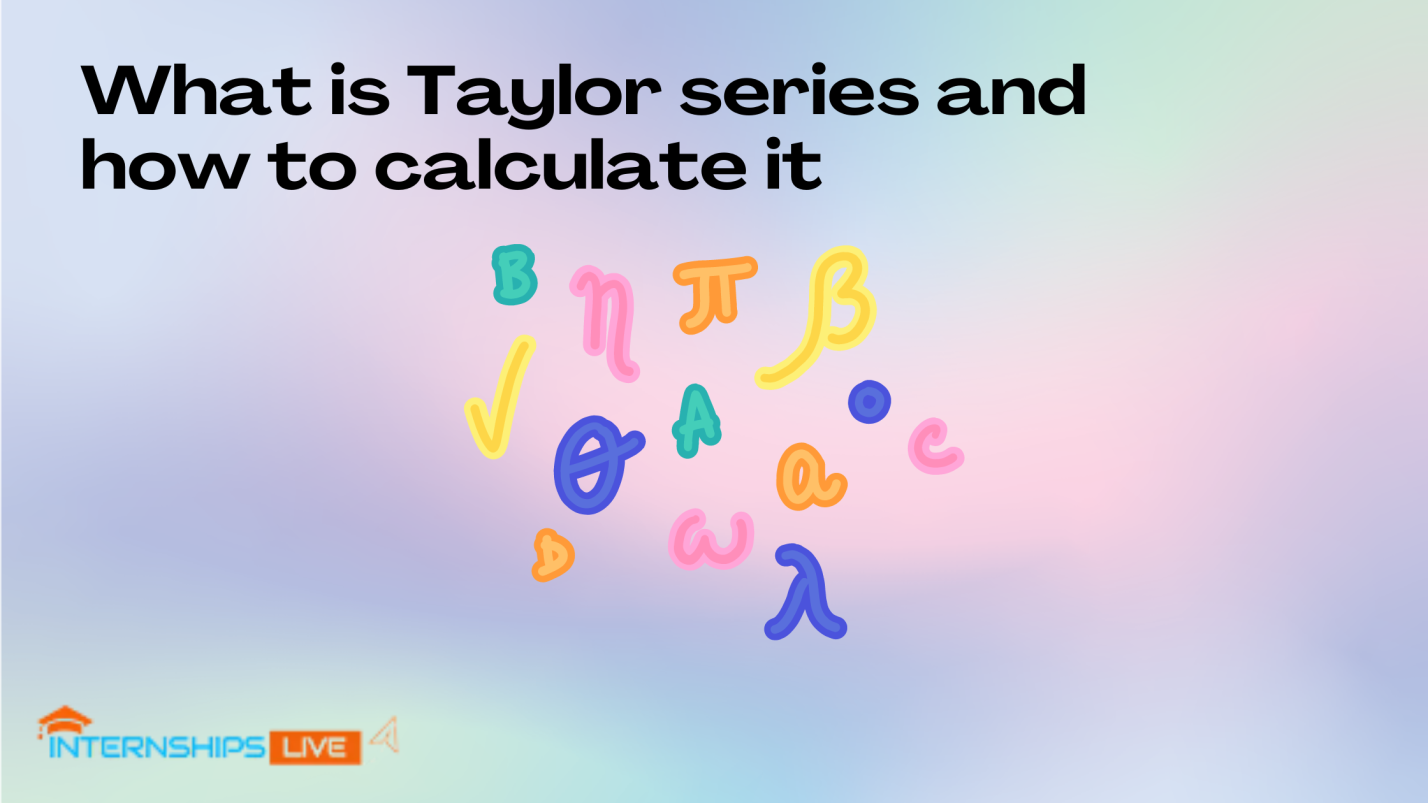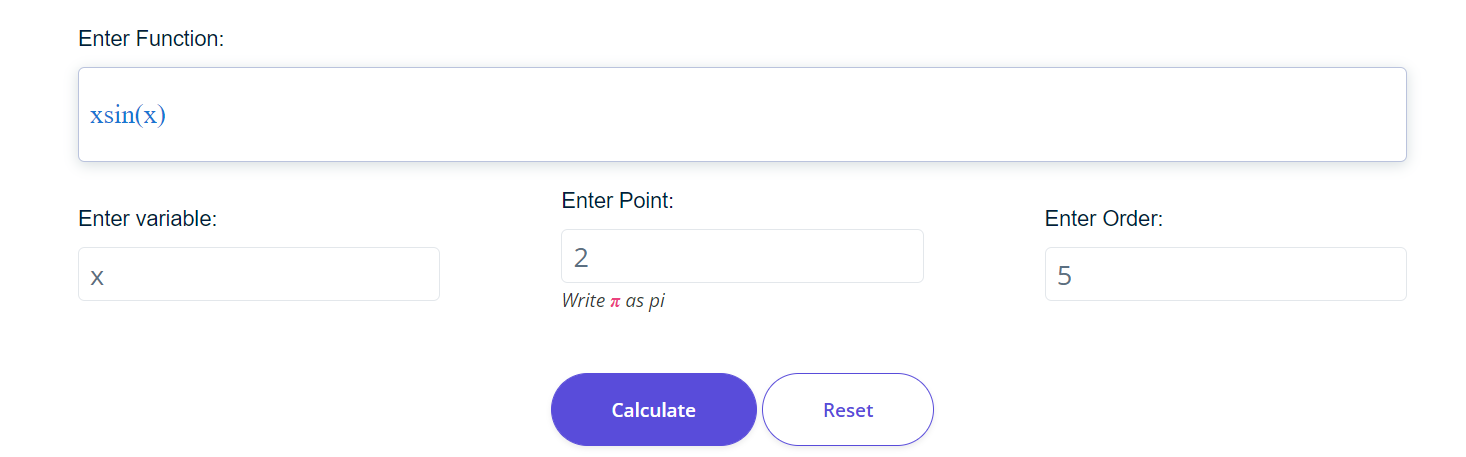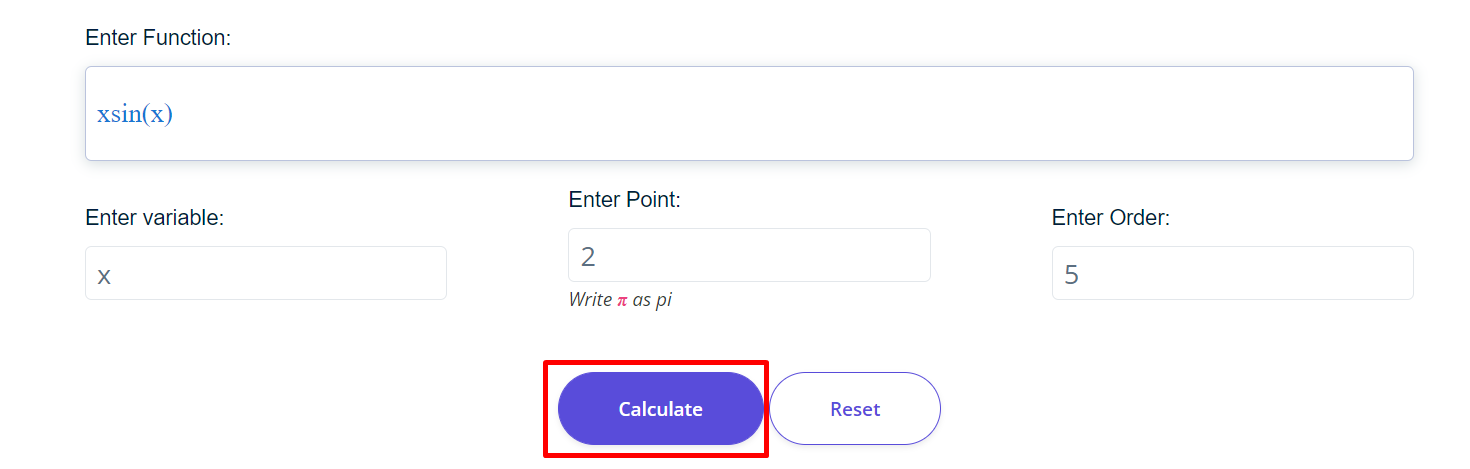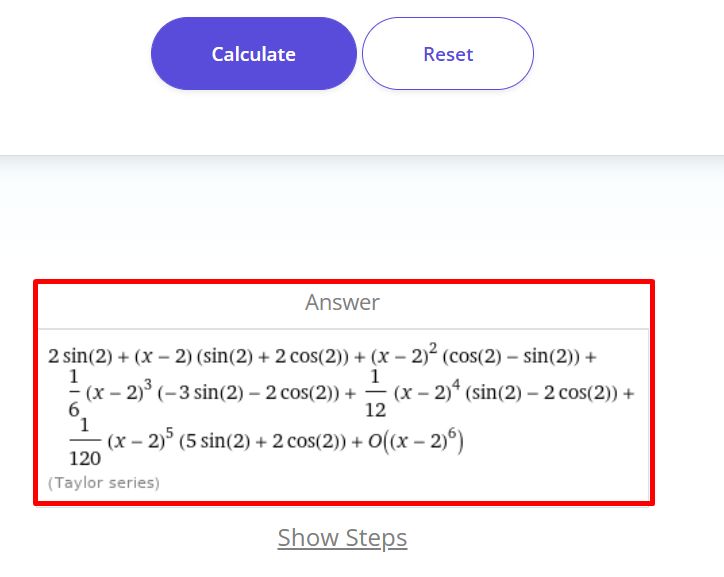# What is the Taylor series and how to calculate it?In mathematics, the Taylor series finds the power series of the given function around the center point up to the nth order of the function. The function can be linear, polynomial, or quadric. In this series, derivatives are used frequently to make the Taylor series.

The differential is widely used in calculus to find the slope of the tangent. In this article, we’ll discuss the Taylor series definition, formula, and how to calculate it.

## What is the Taylor series?

A function of an infinite sum of terms that are expressed in terms of the derivatives of the functions at a single point (center point) is known as the Taylor series. In other words, the Taylor series expands the given function in series around the center point (a) and nth order (n) of the function.

If the derivatives of the function are not existing, then the Taylor series is not existing. For finding the series of the function, all the derivatives must exist and expansion converges to a function. It follows a general equation to expand the given function.

### Equation of the Taylor series

The general formula or equation of the Taylor series is:

F(x) = f(a) + f’(a) (x – a) / 1!  + f’’(a) (x – a)2/ 2!  + f’’’(a) (x – a)3/ 3!  + … + fn(a) (x – a) n/ n!

This formula can also be written in the form of summation.

F(x) = n=0 fn(a) (x – a) n/ n!

• fn(a) is the nth derivative of the function at center point a.
• n is the order of the derivative or total numbers.
• a is the center point.
• F(x) is the series of the given function.

To get the result according to the above formula, you can use a Taylor expansion calculator.

## How to calculate the Taylor series?

Taylor series can be calculated easily just by following the formula of this series. You must be familiar with how to take the derivatives of the function for solving the problems of the Taylor series. Following are a few examples to learn how to calculate the Taylor series.

Example 1

Find the Taylor series of xsin(x) around the center point 2 and the order is 5.

Solution

Step 1: Form the given problem identify the data.

f(x) = xsin(x)

order of the function= n = 5

center point = a = 2

Step 2: Write the general equation to find the Taylor series.

F(x) = n=0 fn(a) (x – a) n/ n!

According to the given order, this equation becomes.

F(x) = n=05 fn(a) (x – a) n/ n!

F(x) = f(a) + f’(a) (x – a) + f’’(a) (x – a)2/2 + f’’’(a) (x – a)3/6 + f’’’’(a) (x – a)4/24 + f’’’’’(a) (x – a)5/120 … (1)

Step 4: Now find the differentials of the given functions.

f(x) = xsin(x)

f’(x) = sin(x) + xcos(x)

f’’(x) = cos(x) + cos(x) + x(-sin(x)) = 2cos(x) – xsin(x)

f’’’(x) = 2(-sin(x)) – sin(x) – xcos(x) = -2sin(x) – sin(x) – xcos(x) = -3sin(x) – xcos(x)

f’’’’(x) = -3cos(x) – cos(x) – x(-sin(x)) = -4cos(x) + xsin(x)

f’’’’’(x) = -4(-sin(x)) + sin(x) + xcos(x) = 4sin(x) + sin(x) + xcos(x) = 5sin(x) + xcos(x)

Step 5: Now open the general equation by putting n = 0, 1, 2,3 ,4, 5 at a.

For n = 0

f(a) (x – a)0/ 0!  = f(a) = asin(a)

For n = 1

f’(a) (x – a)1/ 1!  = f’(a) (x – a) = (sin(a) + acos(a)) (x – a)

For n = 2

f’’(a) (x – a)2/ 2!  = f’’(a) (x – a)2/2 = ½ (2cos(a) – asin(a)) (x – a)2

For n = 3

f’’’(a) (x – a)3/3!  = f’’’(a) (x – a)3/6 = 1 /6 (-3sin(a) – acos(a)) (x – a)3

For n = 4

f’’’’(a) (x – a)4/4!  = f’’’’(a) (x – a)4/24 = 1 /24 (-4cos(a) + asin(a)) (x – a)4

For n = 5

f’’’’’(a) (x – a)5/5! = f’’’’’(a) (x – a)5/120   = 1 /120 (5sin(a) + acos(a)) (x – a)5

Step 6: Now Put the calculated values in (1)

F(x) = f(a) + f’(a) (x – a) + f’’(a) (x – a)2/2 + f’’’(a) (x – a)3/6 + f’’’’(a) (x – a)4/24 + f’’’’’(a) (x – a)5/120 … (1)

F(x) = asin(a) + (sin(a) + acos(a)) (x – a) + ½ (2cos(a) – asin(a)) (x – a)2 + 1 /6 (-3sin(a) – acos(a)) (x – a)3 + 1 /24 (-4cos(a) + asin(a)) (x – a)4 + 1 /120 (5sin(a) + acos(a)) (x – a)5

Step 7: Now replace a = 2 in the above series.

F(x) = 2sin(2) + (sin(2) + 2cos(2)) (x – 2) + ½ (2cos(2) – 2sin(2)) (x – 2)2 + 1 /6 (-3sin(2) – 2cos(2)) (x – 2)3 + 1 /24 (-4cos(2) + 2sin(2)) (x – 2)4 + 1 /120 (5sin(2) + 2cos(2)) (x – 2)5

This is the required expansion of the cos(x) by using the Taylor series

You can verify the result by using a Taylor series calculator. Follow the below steps to use this tool.

Step 1: Write the function, variable, center point a, and total number n into the required boxes.Step 2: Hit the calculate key to get the result.Step 3: The result will show below the calculate button.Example 2

Find the Taylor series of x^3 around the center point 5 and the order is 4.

Solution

Step 1: Form the given problem identify the data.

f(x) = x^3

order of the function= n = 4

center point = a = 5

Step 2: Write the general equation to find the Taylor series.

F(x) = n=0 fn(a) (x – a) n/ n!

According to the given order, this equation becomes.

F(x) = n=04 fn(a) (x – a) n/ n!

F(x) = f(a) + f’(a) (x – a) + f’’(a) (x – a)2/2 + f’’’(a) (x – a)3/6 + f’’’’(a) (x – a)4/24 … (1)

Step 4: Now find the differentials of the given functions.

f(x) = x^3

f’(x) = 3x^2

f’’(x) = 6x

f’’’(x) = 6

f’’’’(x) = 0

Step 5: Now open the general equation by putting n = 0, 1, 2,3 ,4 at a.

For n = 0

f(a) (x – a)0/ 0!  = f(a) = a^3

For n = 1

f’(a) (x – a)1/ 1!  = f’(a) (x – a) = 3a^2 (x – a)

For n = 2

f’’(a) (x – a)2/ 2!  = f’’(a) (x – a)2/2 = ½ 6x (x – a)2 = 3a (x – a)2

For n = 3

f’’’(a) (x – a)3/3!  = f’’’(a) (x – a)3/6 = 1 /6 (6) (x – a)3 = (x – a)3

For n = 4

f’’’’(a) (x – a)4/4!  = f’’’’(a) (x – a)4/24 = 1 /24 (0) (x – a)4 = 0

Step 6: Now Put the calculated values in (1)

F(x) = f(a) + f’(a) (x – a) + f’’(a) (x – a)2/2 + f’’’(a) (x – a)3/6 + f’’’’(a) (x – a)4/24 + f’’’’’(a) (x – a)5/120 … (1)

F(x) = a^3 + 3a^2 (x – a) + 3a (x – a)2 + (x – a)3 + 0

Step 7: Now replace a = 5 in the above series.

F(x) = 5^3 + 3(5^2) (x – 5) + 3(5) (x – 5)2 + (x – 5)3

F(x) = 125 + 75(x – 5) + 15(x – 5)2 + (x – 5)3

## Summary

Now you are witnessed that the Taylor series is not a difficult topic. You can easily solve the problems of the Taylor series just by learning this post.